﻿ 变电站降噪方法研究 Research on Acoustic Noise Reduction Methods for Substation

Open Journal of Acoustics and Vibration
Vol. 06  No. 01 ( 2018 ), Article ID: 23407 , 7 pages
10.12677/OJAV.2018.61001

Research on Acoustic Noise Reduction Methods for Substation

Zheng Dai1, Hugen Wang2, Fei Qiao2, Feng Li1, Yanyuan Qian1, Junsheng Wang2

1State Grid Shanghai Economic Research Institute, SMEPC, Shanghai

2Power China Equipment Research Institute, Shanghai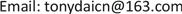Received: Dec. 25th, 2017; accepted: Jan. 8th, 2018; published: Jan. 15th, 2018ABSTRACT

This paper discusses the use of perforated plate resonant sound absorption structure for substation noise control. Based on the noise distribution characteristics of the substation, the resonant sound absorption structure parameters are designed, and the sound absorption coefficient of the resonant structure is numerically simulated. It is shown that the structure has a relatively large sound absorption coefficient at low frequency. Experiments are conducted to verify the perforated plate resonant sound absorption structure and a software is designed to obtain the substation audible noise frequency A weighted sound pressure level. The results show that the resonant sound absorption structure of the perforated plate has better frequency selection sound absorption characteristics, which greatly reduces the low frequency noise in the substation and makes the noise of the substation get effective control.

Keywords:Resonance Absorbing, Perforated Panel Structure, Noise Frequency Distribution, Substation Noise Control, Sound Pressure Level

1国网上海市电力公司经济技术研究院，上海

2中国电建集团装备研究院有限公司，上海1. 引言

2. 穿孔板共振吸声原理

$\alpha =\frac{\text{4}r}{{\left(1+r\right)}^{2}+{\left[\omega m-\mathrm{cot}\left(\frac{\omega D}{{c}_{0}}\right)\right]}^{2}}$ (1)

$r=\frac{32\eta t}{q{c}_{0}{\rho }_{0}{d}^{2}}\left[{\left(1+\frac{{|Ka|}^{2}}{32}\right)}^{1/2}+\frac{\sqrt{2}}{32}\frac{|Ka|d}{t}\right]$ (2)

$m=\frac{\left(t+0.85d\right)}{q{c}_{0}}\left[1+{\left(9+\frac{{|Ka|}^{2}}{2}\right)}^{-1/2}\right]$ (3)

$|Ka|=\frac{d}{2}\sqrt{\frac{\omega {\rho }_{0}}{\eta }}$ (4)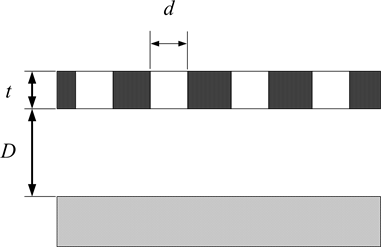Figure 1. The structure of the perforated panel

${L}_{p}=10\mathrm{lg}\frac{{P}^{2}}{{P}_{0}^{2}}=20\mathrm{lg}\frac{P}{{P}_{0}}$ (5)

P0为参考声压值，为2 × 105 (Pa)。根据上式可得到噪声的声压级，但人耳对不同频率的噪声具有不同的感觉，因此需要对声压级信号进行计权。常用的计权网络有A、B、C、D计权。

A计权网络是模仿40方等响曲线设计的。当声波通过A计权网络时，低频声(特别是500 Hz以下)衰减较大，而对1000~5000 Hz的声音不衰减，甚至稍有放大，B计权是仿效70方等响曲线，低频有衰减，C计权是仿效100方等响曲线，有近似平直的响应，D计权网络是为测量飞机噪声而设计的。实践证明，A计权网络比较接近人耳对声音的感觉特性，所以在噪声测量仪器〈例如声级计〉中普遍采用A计权。然后把得到的声压级信号进行A计权网络修正得到可听噪声A计权声压级。所测得的声级就称作A声级、记作dB (A)。

${L}_{{p}_{i}}=10\mathrm{lg}\left(\frac{{P}_{i}^{2}}{{P}_{0}^{2}}\right)$ (6)

$\left(\frac{{P}_{i}^{2}}{{P}_{0}^{2}}\right)={10}^{\frac{{L}_{{p}_{i}}}{10}}$ ，所有n个倍频带声压级叠加后总声压级Lp为：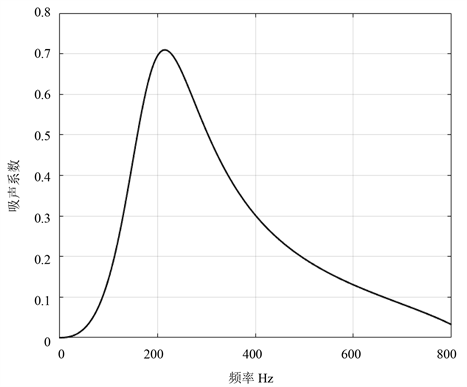Figure 2. The relationship between the acoustic absorbing coefficients of the perforated panel and the frequency

${L}_{p}=10\mathrm{lg}\left[\underset{i=1}{\overset{10}{\sum }}{10}^{\frac{{L}_{{p}_{i}}}{10}}\right]$ (7)

${L}_{{p}_{A}}=10\mathrm{lg}\left[\underset{i=1}{\overset{n}{\sum }}{10}^{\frac{{L}_{{p}_{i}}-{K}_{i}}{10}}\right]$ (8)

3. 工程实例

4. 结论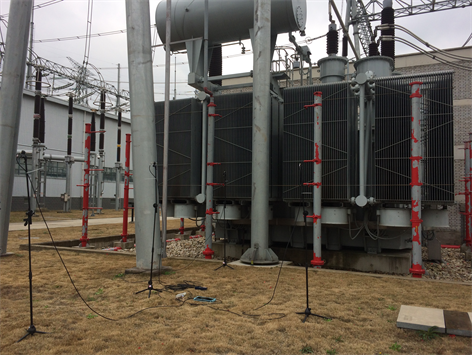Figure 3. Sound pressure level test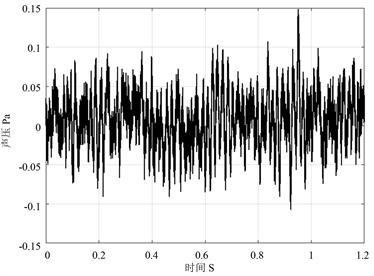Figure 4. The acoustic pressure of acquired signals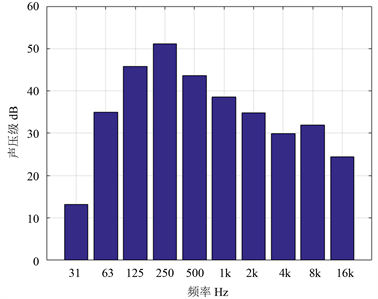Figure 5. A-weighted acoustic pressure level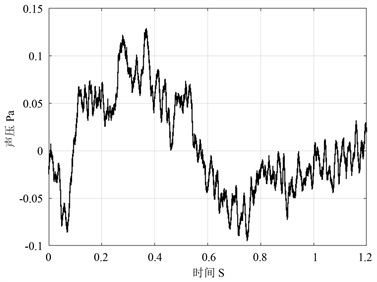Figure 6. The acquired acoustic signals after installed an acoustic absorbing structure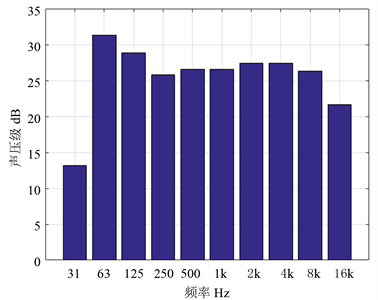Figure 7. The A-weight acoustic pressure level of the signal after installed an acoustic absorbing structure

 欧炎, 高聚元. 大型变电站噪声综合治理设计[J]. 噪声与振动控制, 2005(S1): 20-23.

 袁聪波, 黄家彬. 变电站噪声分析及治理[J]. 上海电力, 2002(4): 59-61.

 黄青青, 冯苗锋, 王庭佛. 220 kV变压器噪声及其传播特性浅析[J]. 环境工程, 2011, 29(s1): 330-333.

 周新祥. 噪声控制及应用实例[M]. 北京: 海洋出版社, 1999.

 马大猷. 现代声学理论基础[M[. 北京: 科学出版社, 2004.

 牛磊. 电力变压器噪声实验室测量方法的研究[D]: [硕士学位论文]. 合肥: 合肥工业大学, 2013.